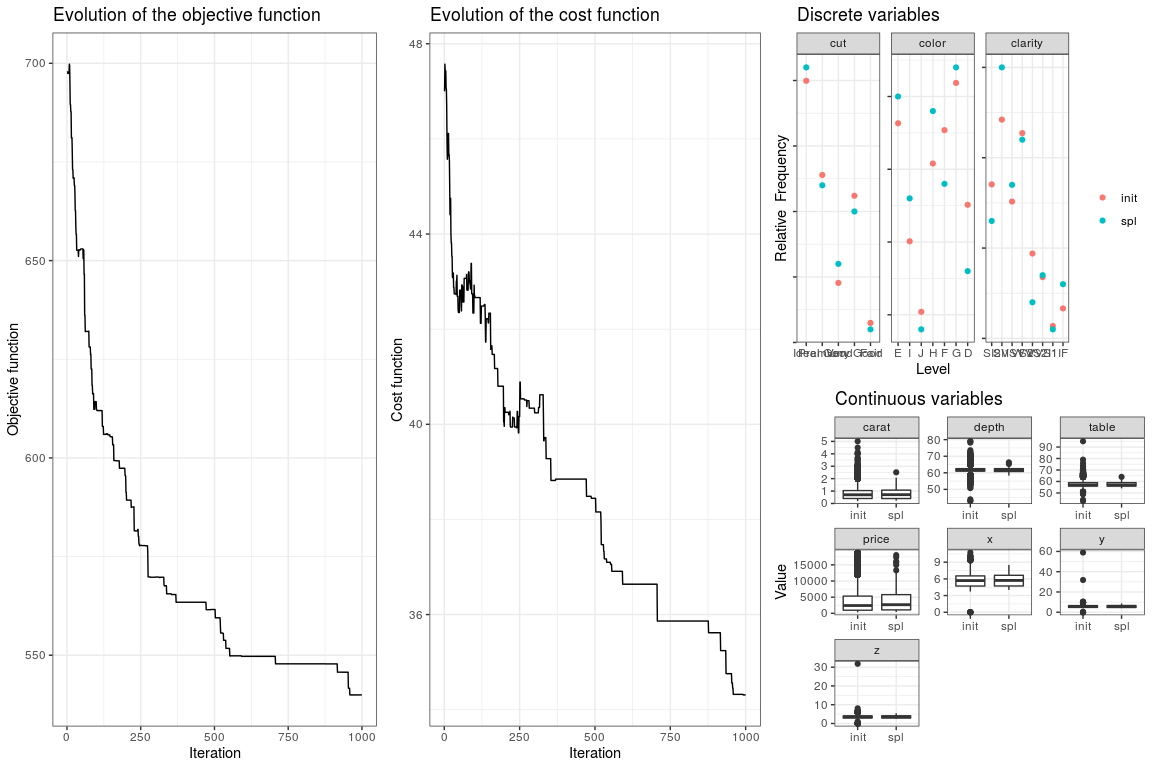# A simple example

data(diamonds, package = 'ggplot2')
diamonds <- data.frame(diamonds)
head(diamonds)
##   carat       cut color clarity depth table price    x    y    z
## 1  0.23     Ideal     E     SI2  61.5    55   326 3.95 3.98 2.43
## 2  0.21   Premium     E     SI1  59.8    61   326 3.89 3.84 2.31
## 3  0.23      Good     E     VS1  56.9    65   327 4.05 4.07 2.31
## 4  0.29   Premium     I     VS2  62.4    58   334 4.20 4.23 2.63
## 5  0.31      Good     J     SI2  63.3    58   335 4.34 4.35 2.75
## 6  0.24 Very Good     J    VVS2  62.8    57   336 3.94 3.96 2.48
nrow(diamonds)
##  53940

In this example we sample the diamonds data set and pick a subset of 100 individuals using the cLHS method. To reduce the length of the optimisation step to 1000 iterations to save computing time. This is controlled through the iter option. The progress bar is disabled because it doesn’t renders well in the vignette. By default, the index of the selected individuals in the original object are returned.

library(clhs)
res <- clhs(diamonds, size = 100, progress = FALSE, iter = 1000)
str(res)
##  int [1:100] 44792 35433 24687 38782 50406 25613 30214 48746 7480 53320 ...

# Tweaking the parameters

(work in progress)

# Including existing samples in the sampling routine

This functionality is controlled by the include option, which can be used to specify the row indices oif samples that needs to be included in the final sampled set.

suppressWarnings(RNGversion("3.5.0"))
set.seed(1)

candidates_samples <- data.frame(
x = runif(500),
y = rnorm(500, mean = 0.5, sd = 0.5)
)

existing_samples <- data.frame(
x = runif(5),
y = runif(5)
)

res <- clhs(
x = rbind(existing_samples, candidates_samples),
size = 10,
iter = 100,
include = 1:5,
progress = FALSE
)

In this case we have 5 individuals (red triangles) that need to be retained in the selected set of samples:

## Warning in (function (kind = NULL, normal.kind = NULL, sample.kind = NULL) :
## non-uniform 'Rounding' sampler used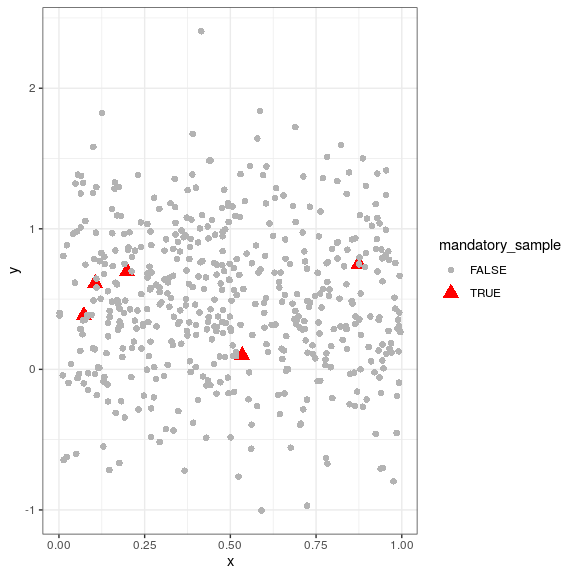The red individuals are the selected samples. Note the triangles showing the samples that were compulsory: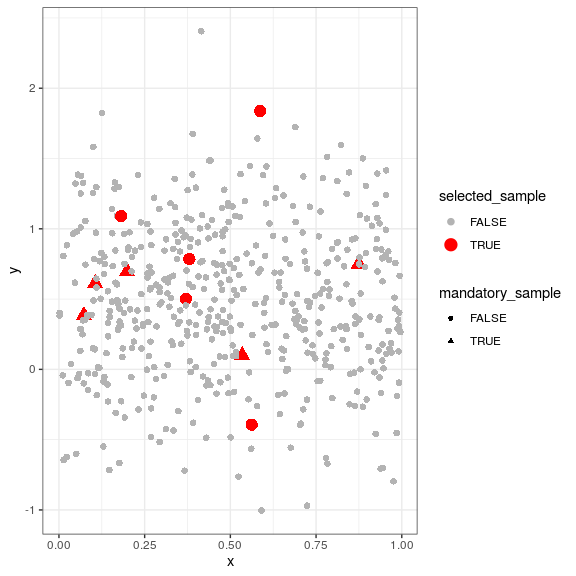# Cost-constrained implementation

(work in progress)

diamonds\$cost <- runif(nrow(diamonds))
res_cost <- clhs(diamonds, size = 100, progress = FALSE, iter = 1000, cost = 'cost')

# DLHS

(work in progress)

# Plotting the results

If you want to report on the cLHS results, e.g. plot the evolution of the objective function, or compare the distribution of attributes in the initial object and in the sampled subset, you need to switch the simple option to FALSE. Instead f simply returning a numeric vector giving the index of the sampled individuals in the original object, a specific, more complex will be returned. This object can be handled by a specific plot method:

res <- clhs(diamonds, size = 100, simple = FALSE, progress = FALSE, iter = 1000)
plot(res)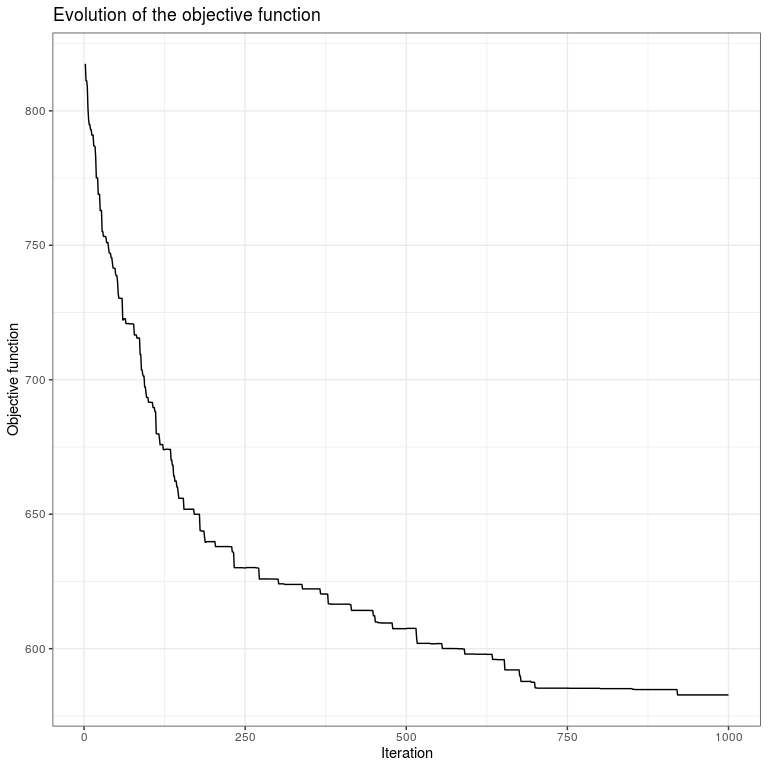The default plotting method plots the evolution of the objective function with the number of iterations. However, you can get more details using the modes option, which controls which indicators are plotted. Three modes can be simultaneously plotted:

• obj: evolution of the objective function (default)
• cost: evolution of the cost function (if present)
• dens OR box OR hist: comparison of the distributions of each attribute in the original object and in the proposed sample, respectively using probability density functions, boxplots or histograms. Note that categorical attributes are always reported using dotplots.

These modes should be given as a vector of characters.

res_cost <- clhs(diamonds, size = 100, progress = FALSE, iter = 1000, cost = 'cost', simple = FALSE)
plot(res_cost, c('obj', 'cost'))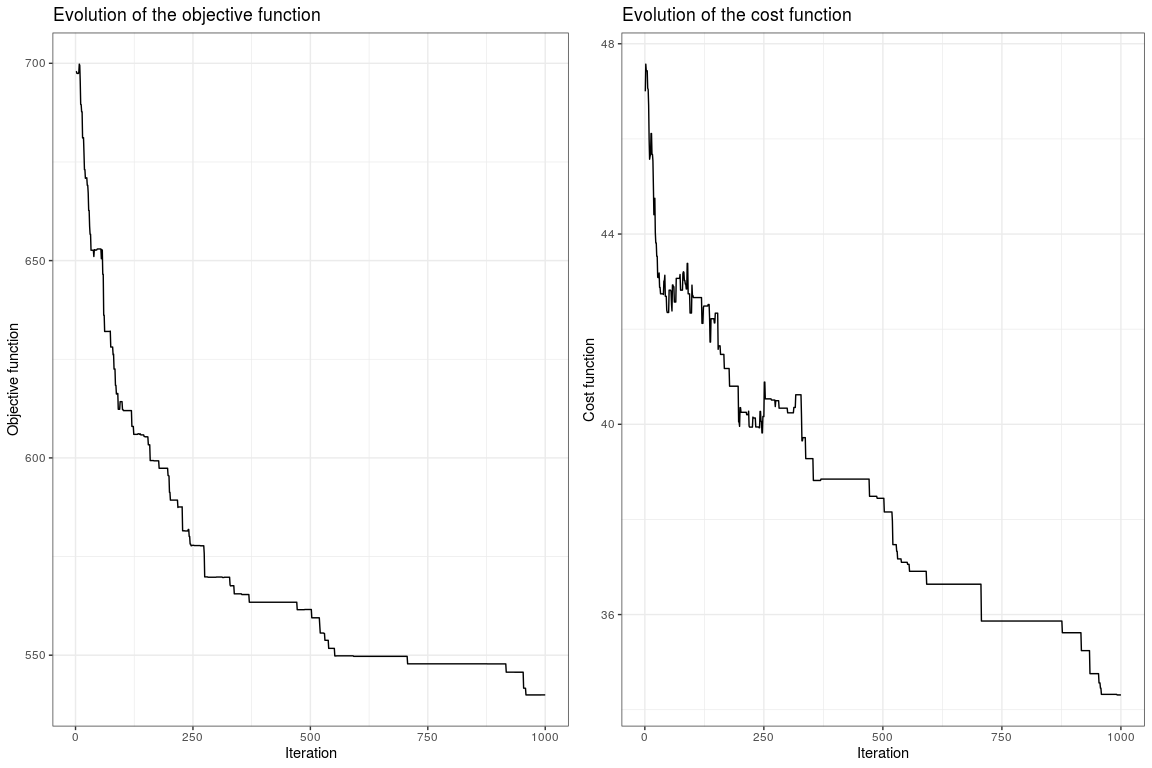plot(res_cost, c('obj', 'cost', 'box'))